# Rational function

(diff) ← Older revision | Latest revision (diff) | Newer revision → (diff)
Jump to: navigation, search

A rational function is a function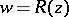, where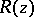is rational expression in, i.e. an expression obtained from an independent variableand some finite set of numbers (real or complex) by means of a finite number of arithmetical operations. A rational function can be written (non-uniquely) in the form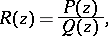where,are polynomials,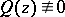. The coefficients of these polynomials are called the coefficients of the rational function. The functionis called irreducible whenandhave no common zeros (that is,andare relatively prime polynomials). Every rational function can be written as an irreducible fraction; ifhas degreeandhas degree, then the degree ofis either taken to be the pair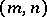or the number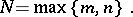A rational function of degreewith, that is, a polynomial, is also called an entire rational function. Otherwise it is called a fractional-rational function. The degree of the rational function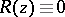is not defined. When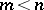, the fraction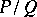is called proper, and it is called improper otherwise. An improper fraction can be uniquely written as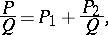whereis a polynomial, called the integral part of the fraction, and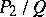is a proper fraction. A proper fraction,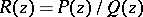, in irreducible form, where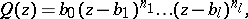admits a unique expansion as a sum of simple partial fractions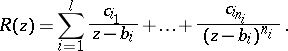(1)

If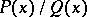is a proper rational function with real coefficients andwhere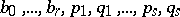are real numbers such that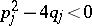for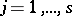, thencan be uniquely written in the form(2)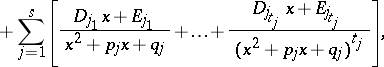where all the coefficients are real. These coefficients, like the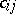in (1), can be found by the method of indefinite coefficients (cf. Undetermined coefficients, method of).

A rational function of degreein irreducible form is defined and analytic in the extended complex plane (that is, the plane together with the point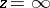), except at a finite number of singular points, poles: the zeros of its denominator and, when, also the point. Note that if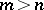, the sum of the multiplicities of the poles ofis equal to its degree. Conversely, ifis an analytic function whose only singular points in the extended complex plane are finitely many poles, thenis a rational function.

The application of arithmetical operations (with the exception of division by) to rational functions again gives a rational function, so that the set of all rational functions forms a field. In general, the rational functions with coefficients in a field form a field. If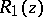,are rational functions, then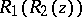is also a rational function. The derivative of orderof a rational function of degreeis a rational function of degree at most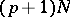. An indefinite integral (or primitive) of a rational function is the sum of a rational function and expressions of the form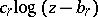. If a rational function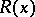is real for all real, then the indefinite integral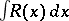can be written as the sum of a rational functionwith real coefficients, expressions of the form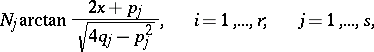and an arbitrary constant(where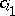,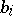,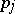,are the same as in (2), and,are real numbers). The functioncan be found by the Ostrogradski method, which avoids the need to expandinto partial fractions (2).

For ease of computation, rational functions can be used to approximate a given function. Attention has also been paid to rational functions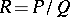in several real or complex variables, whereandare polynomials in these variables with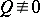, and to abstract rational functions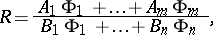where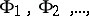are linearly independent functions on some compact space, and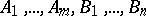are numbers. See also Fractional-linear function; Zhukovskii function.